# How to count specific words in a cell or a range in Excel

This post explains that how to count the number of a specific word in a cell or a range of cells in excel. This post will guide you how to count specific words in a single cell or a range using excel formula. How to count the occurrence of a specific word in a cell or a range of cells in excel.

## Count specific words in a single cell

If you want to count the number of a specific word in a single cell, you need to use the SUBSTITUTE function to remove all that certain word in text string, then using LEN function to calculate the length of the substring that without that specific word. Then using the length value of the original text string subtract the length of the substring that without that specific word. So that you can get the length of all that specific words in a single cell. Then the result is divided by the length of that specific word, so that you can get the numbers of that word in that single cell.

Based on the above analysis, we can write down the following excel formula:

`= (LEN(B1)-LEN(SUBSTITUTE(B1,"excel",""))) / LEN("excel")`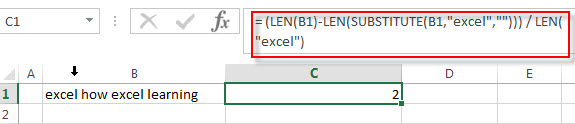Let’s see how this formula works:

= LEN(“excel”)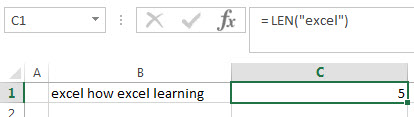This formula will calculate the length of a specific word “excel”.

=LEN(SUBSTITUTE(B1,”excel”,””))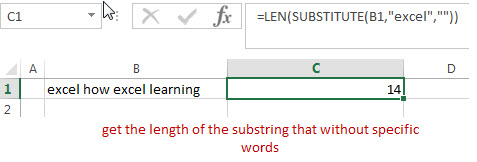The SUBSTITUTE function will replace all “excel” substring with new_text empty string. Then the returned value goes into the LEN function to get the length of the substring that without specific words.

=(LEN(B1)-LEN(SUBSTITUTE(B1,”excel”,””)))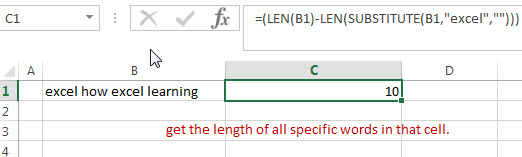This formula will get the length of all specific words in that cell.

## Count specific words in a range of cells

The above formula can only count the number of specific word in a single cell and how to count the number of certain word in a range of cells, you can use the SUMPRODUCT function to sum the number of certain word for each cell in a range, so you can write down the following formula:

`=SUMPRODUCT((LEN(B1:B3)-LEN(SUBSTITUTE(B1:B3,"excel",""))) / LEN("excel"))`

Let’s see how this formula works:

=(LEN(B1:B3)-LEN(SUBSTITUTE(B1:B3,”excel”,””))) / LEN(“excel”)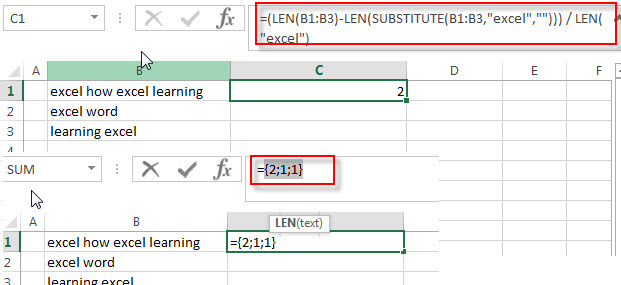This formula will return an array result that contains a number of certain word for each cell in a range B1:B3.the array result like this: {2;1;1} Then the returned array goes into the SUMPRODUCT function as its argument.

=SUMPRODUCT()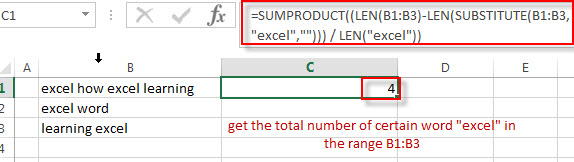This formula will sum all items in that array result that returned by the above formula to get the total number of certain word “excel” in the range B1:B3.

### Related Formulas

• Count the number of words in a cell
If you want to count the number of words in a single cell, you can create an excel formula based on the IF function, the LEN function, the TRIM function and the SUBSTITUTE function. ..
• Extract word that starting with a specific character
Assuming that you have a text string that contains email address in Cell B1, and if you want to extract word that begins with a specific character “@” sign, you can use a combination with the TRIM function, the LEFT function, the SUBSTITUTE function, the MID function, the FIND function, the LEN function and the REPT function to create an excel formula.…

### Related Functions

• Excel Substitute function
The Excel SUBSTITUTE function replaces a new text string for an old text string in a text string.The syntax of the SUBSTITUTE function is as below:= SUBSTITUTE  (text, old_text, new_text,[instance_num])….
• Excel SUMPRODUCT function
The Excel SUMPRODUCT function multiplies corresponding components in the given one or more arrays or ranges, and returns the sum of those products.The syntax of the SUMPRODUCT function is as below:= SUMPRODUCT (array1,[array2],…)…
• Excel LEN function
The Excel LEN function returns the length of a text string (the number of characters in a text string).The syntax of the LEN function is as below:= LEN(text)…